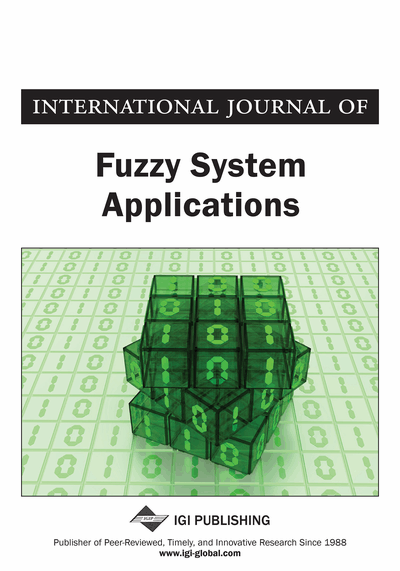# Percentile Matching Estimation of Zigzag Uncertainty Distribution

S. Sampath (Department of Statistics, University of Madras, Chennai, India) and K. Anjana (University of Madras, Chennai, India)
DOI: 10.4018/IJFSA.2018010105

## Abstract

The problem of estimating parameters involved in zigzag uncertainty distribution is considered in this article. Sensing the difficulties involved in the direct application of statistical estimation techniques for uncertainty distribution, the present article considers the application of the method of percentile matching for estimating the unknown parameters of zigzag uncertainty distribution. This article clearly establishes the fact that the percentile matching method gives better estimates when compared to the method of moments if sample percentiles of appropriate orders are used in the estimation process. Detailed numerical studies have been carried out using simulated datasets possessing different characteristics for identifying optimal orders of percentiles which give better estimates of parameters.
Article Preview
Top

## 1. Introduction

Different types of uncertainties arise in real life situations. According to Liu (2008) randomness and impreciseness (fuzziness) are basic types of objective uncertainty and subjective uncertainty, respectively. Probability theory has been developed to handle random phenomena in which the events are well defined and considered not to have vagueness or uncertainty. The idea of fuzzy set theory has been introduced by Zadeh (1965) in order to deal with fuzziness through membership values. Later the concept of fuzzy graphs has been introduced by Rosenfield (1975). Fuzzy robust graph coloring problem has been discussed in Dey, Pradhan, Pal and Pal (2015). Vertex coloring of a fuzzy graph using alpha cut can be seen in Dey and Pal (2012). Interval type 2 fuzzy set in fuzzy shortest path problem is available in Dey, Pal and Pal (2016). Several works on the applications of fuzzy set theory have been carried out in different branches of statistics.

Liu (2007) introduced the concept of Uncertainty theory. According to Liu (2017), the concept of uncertainty theory is one of the options available to deal with indeterminate phenomena whose outcomes cannot be predicted in advance. It turned out to be the solution for problems in the contexts where no samples are available which creates difficulty in using probability theory for dealing with such situations. In such cases, opinions of the domain experts become the only choice for further study. Liu (2017) framed uncertainty theory to model the belief degrees of domain experts in various contexts. Belief degree refers to the belief levels of experts regarding the occurrence of particular events. Structural characteristics of uncertain measure have been discussed in Zhang (2011). One can find similarities in the process of developing uncertainty theory with that of probability theory. However, the ideas developed in uncertainty theory find applications in dealing with problems arising out of impreciseness created in non-stochastic manner.

Liu (2017) pioneered the uncertainty theory over the years and has developed several study areas similar to that existing in probability theory. Uncertain Measure, Uncertain Variable, Uncertain Programming, Uncertain Risk Analysis, Uncertain Reliability Analysis, Uncertain Propositional Logic, Uncertain Set, Uncertain Logic, Uncertain Inference, Uncertain Process, Uncertain Calculus, Uncertain Differential Equation, Uncertain Finance and Uncertain Statistics are some of the concepts developed under uncertainty theory. Liu (2017) gives a detailed explanation of these concepts. Studies on testing uncertain hypotheses about uncertainty distribution functions have been made by Wang, Gao and Guo (2012) and Sampath and Ramya (2013). The concept of uncertain random variables has been introduced by Liu (2013) as a mixture of uncertainty and randomness.

## Complete Article List

Search this Journal:
Reset
Open Access Articles: Forthcoming
Volume 9: 4 Issues (2020): 1 Released, 3 Forthcoming
Volume 8: 4 Issues (2019)
Volume 7: 4 Issues (2018)
Volume 6: 4 Issues (2017)
Volume 5: 4 Issues (2016)
Volume 4: 4 Issues (2015)
Volume 3: 4 Issues (2013)
Volume 2: 4 Issues (2012)
Volume 1: 4 Issues (2011)
View Complete Journal Contents Listing